# Frank Solutions for Class 9 Maths Chapter 11 Triangles and Their Congruency

Frank Solutions for Class 9 Maths Chapter 11 Triangles and Their Congruency contain answers in a step-by-step manner, as per the student’s understanding abilities. Students who aspire to enhance their problem-solving skills are suggested to practise these solutions on a daily basis, which will help them excel in the annual examination with good marks.

Chapter 11 has problems based on determining congruent triangles, as per the current ICSE Board syllabus. Students can refer to these solutions to clear their doubts during the self-study process. The main purpose of providing the solutions is to help students boost their exam preparation. They can download Frank Solutions for Class 9 Maths Chapter 11 Triangles and Their Congruency from the link provided below.

## Frank Solutions for Class 9 Maths Chapter 11 Triangles and Their Congruency Download Free PDF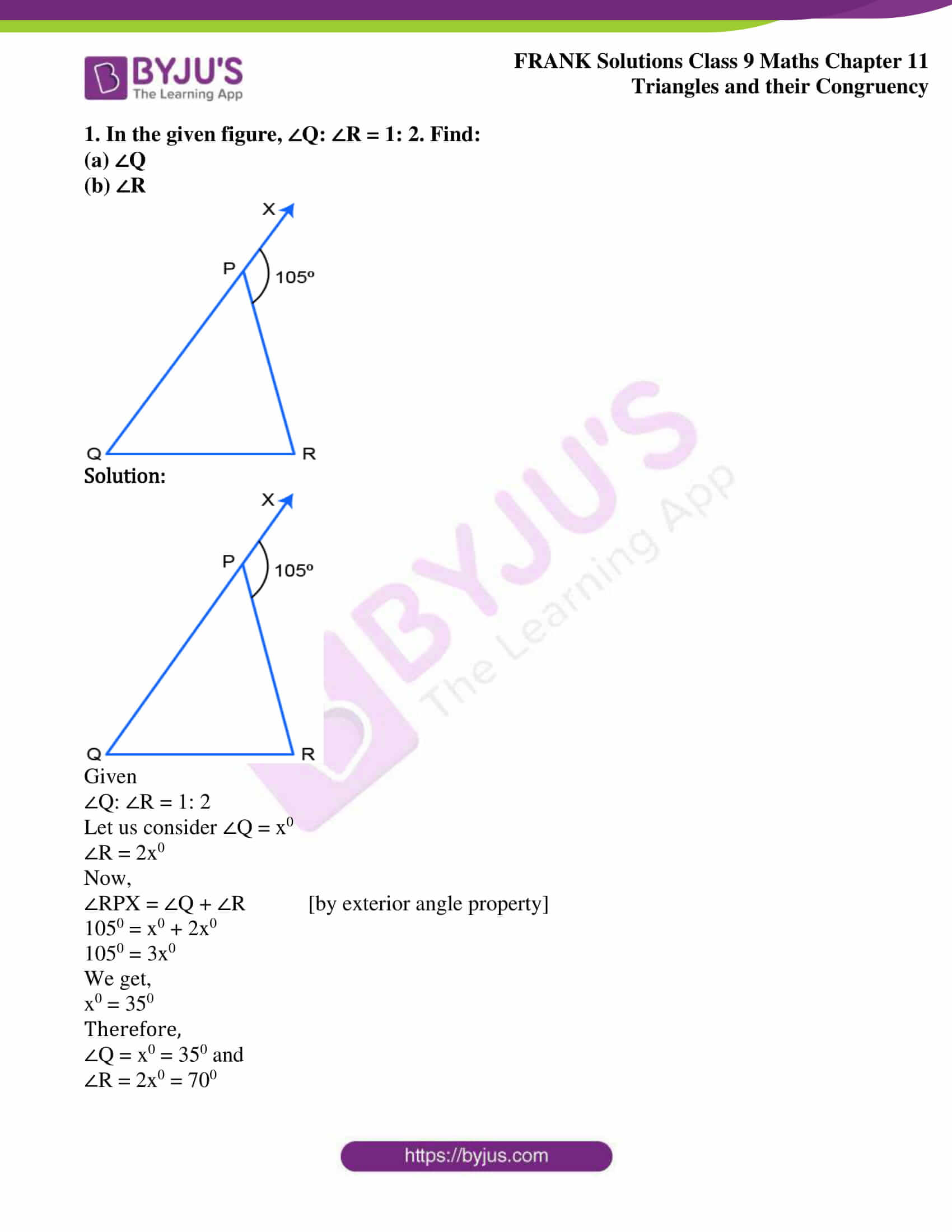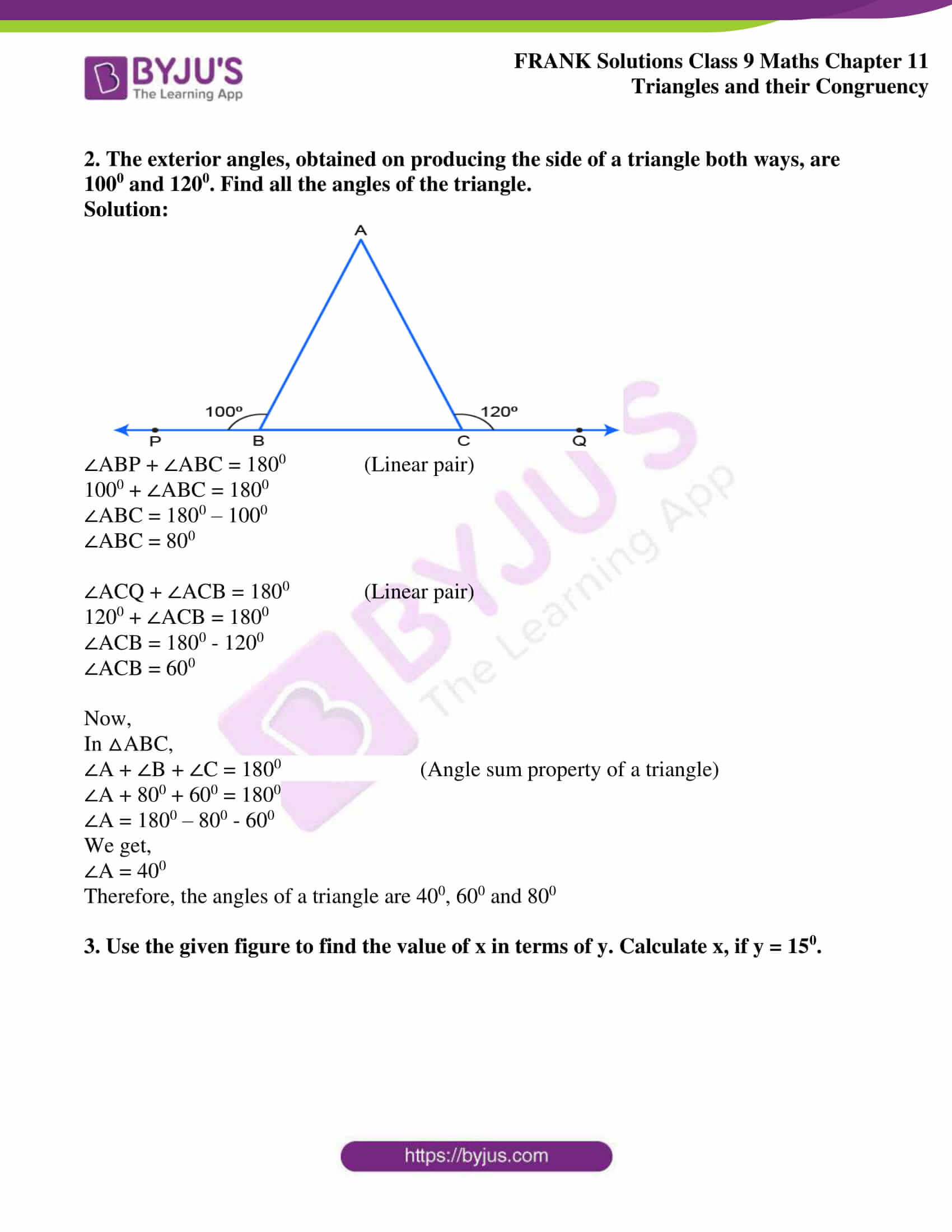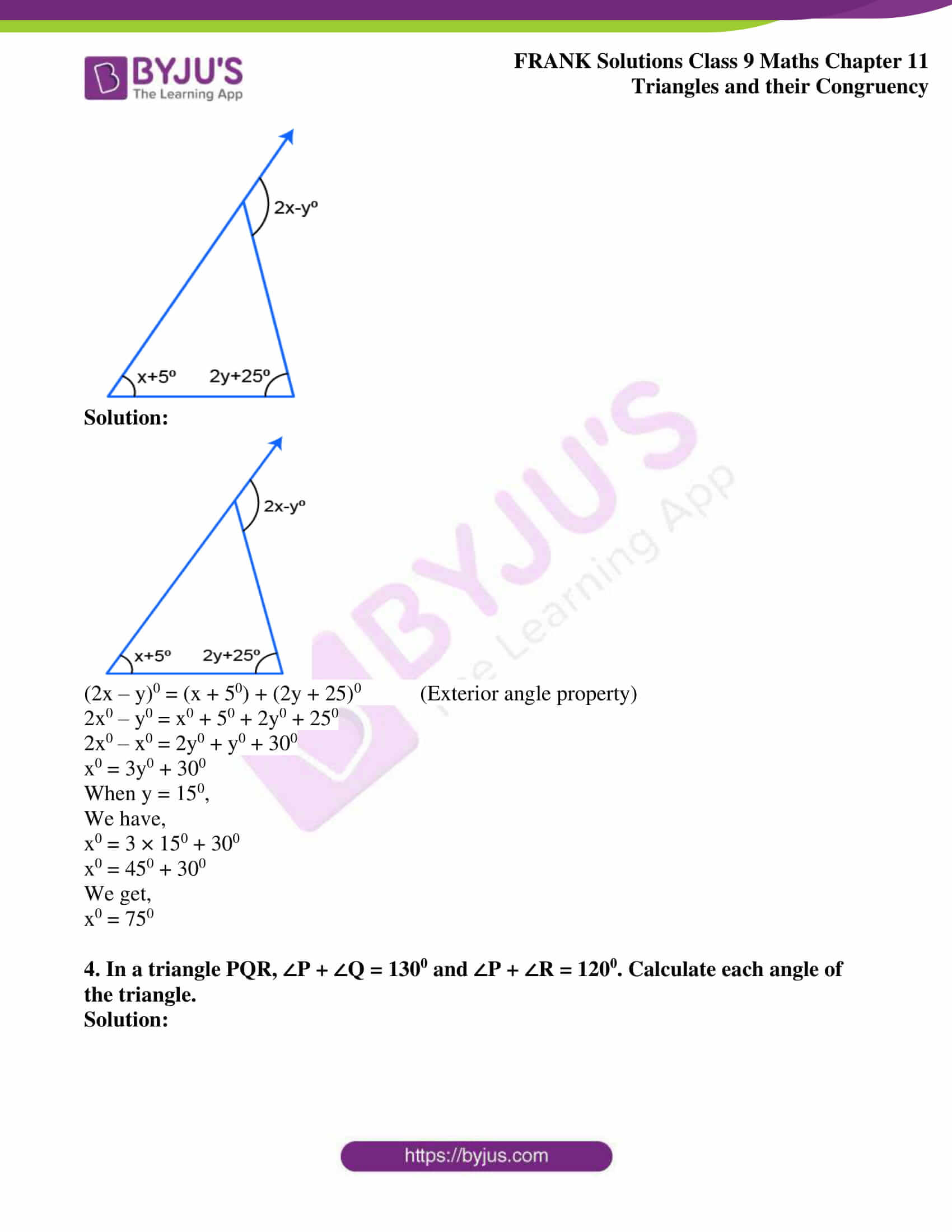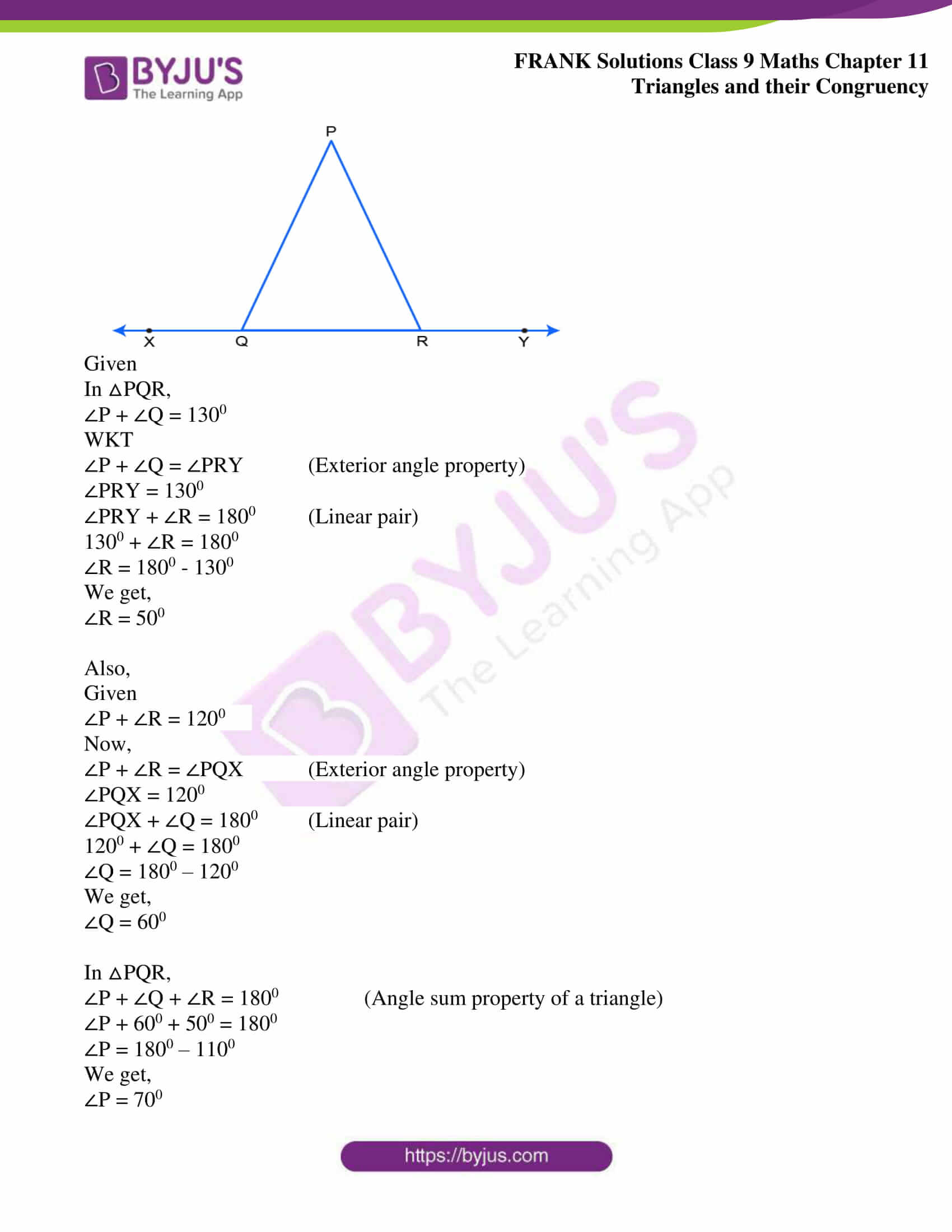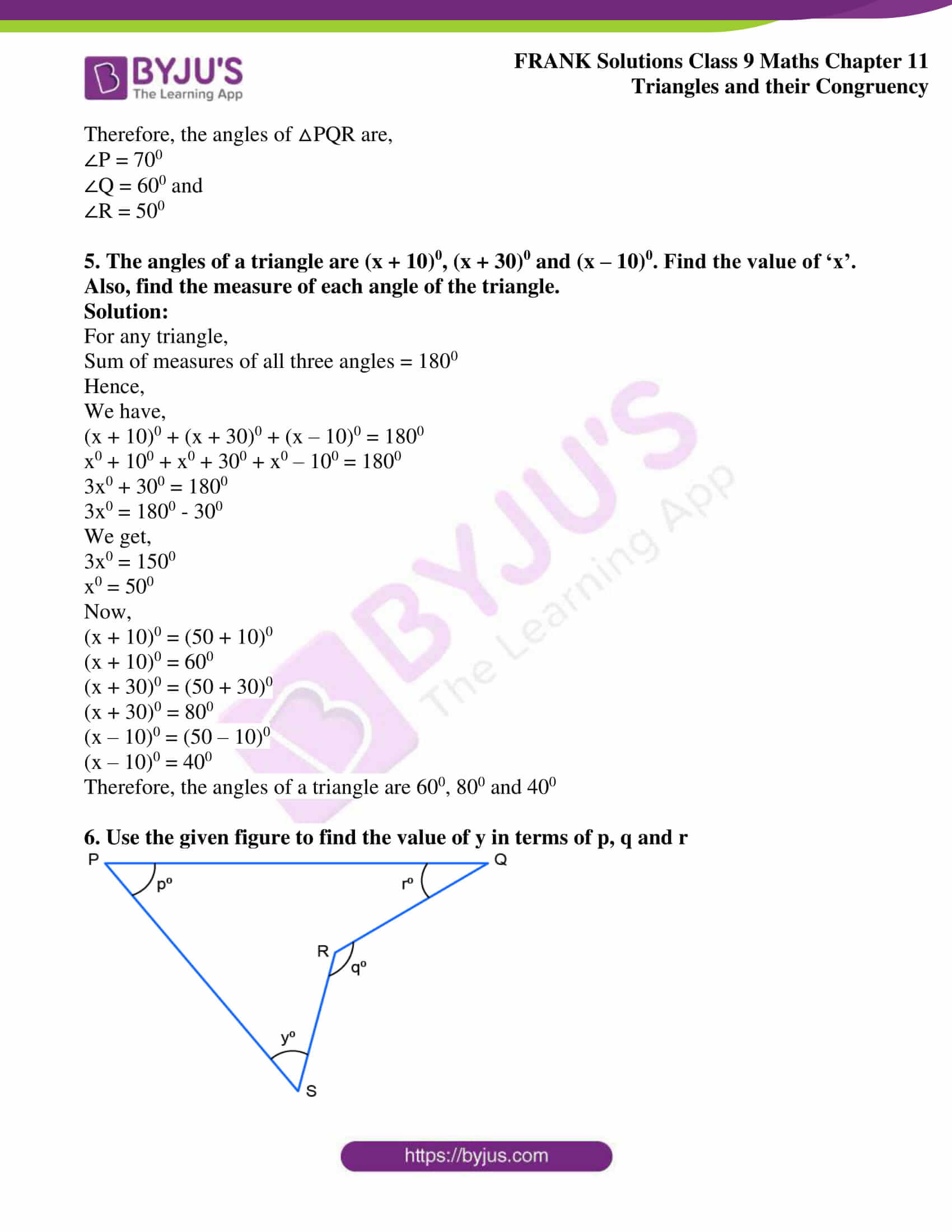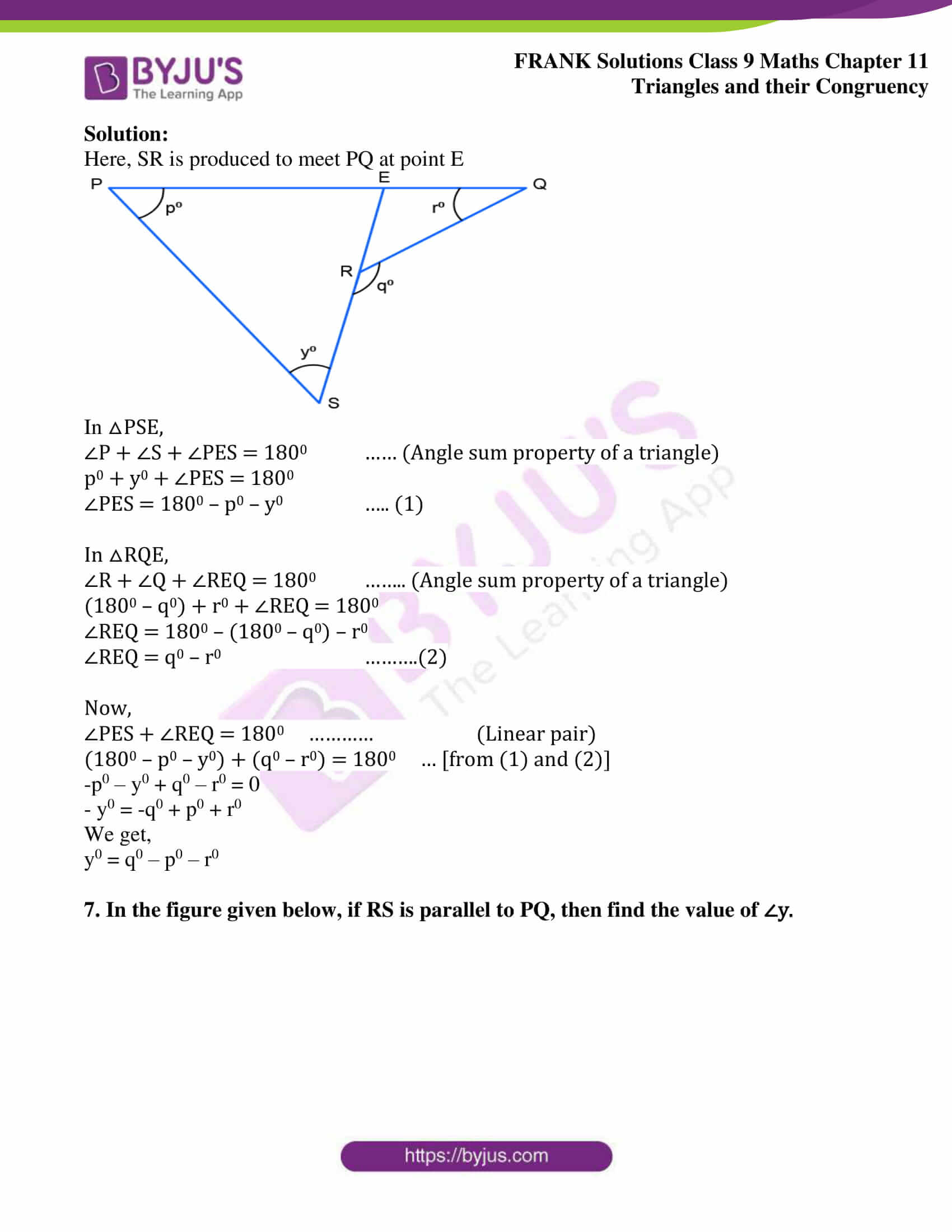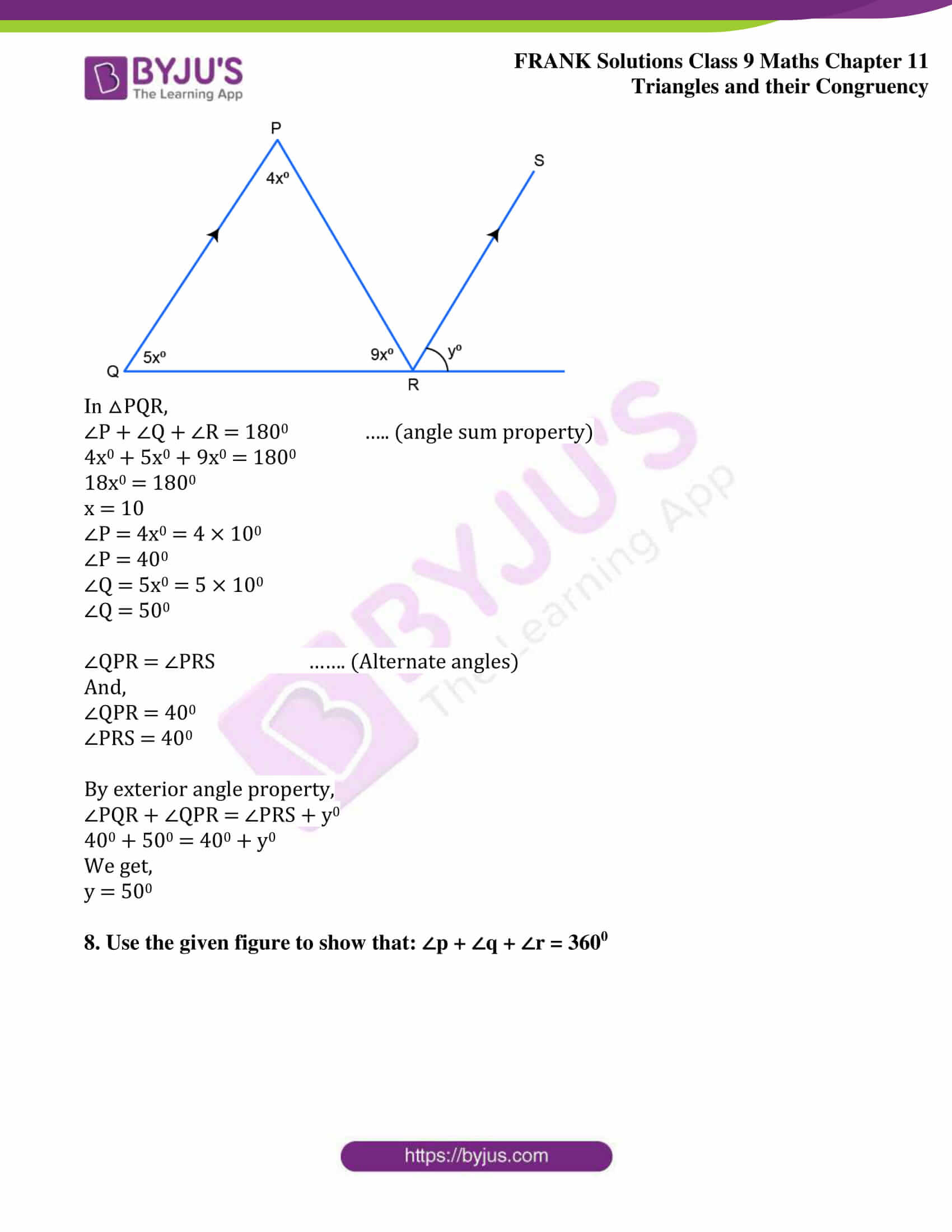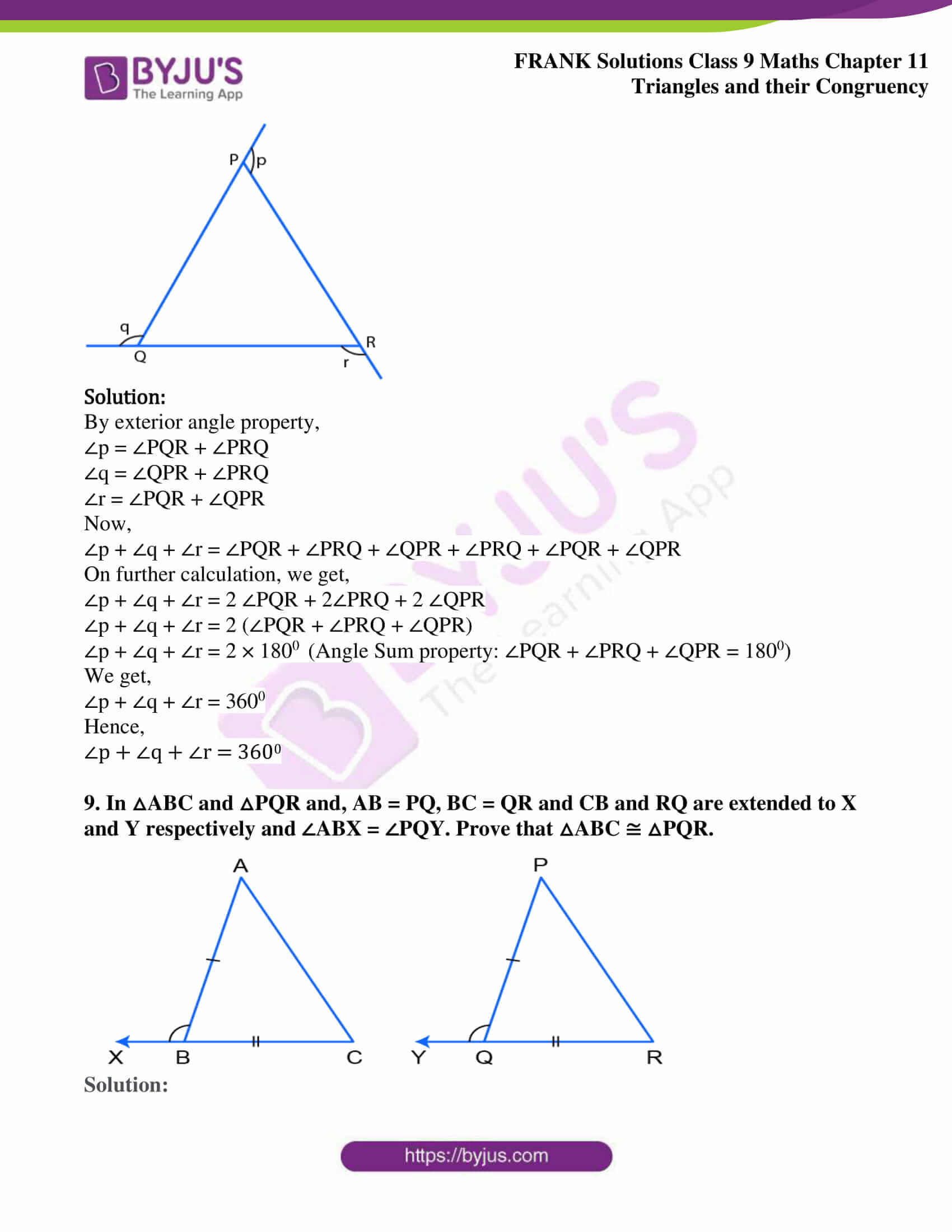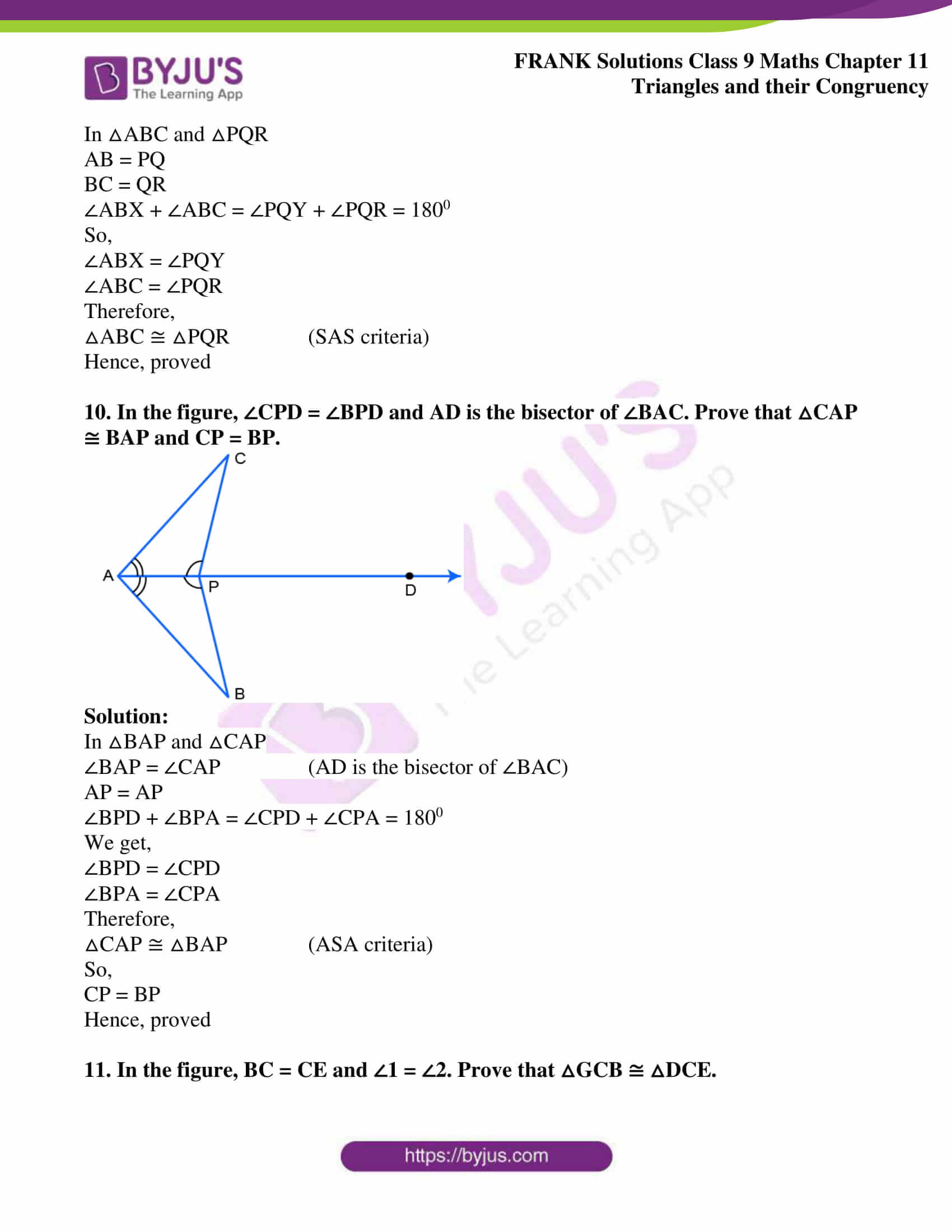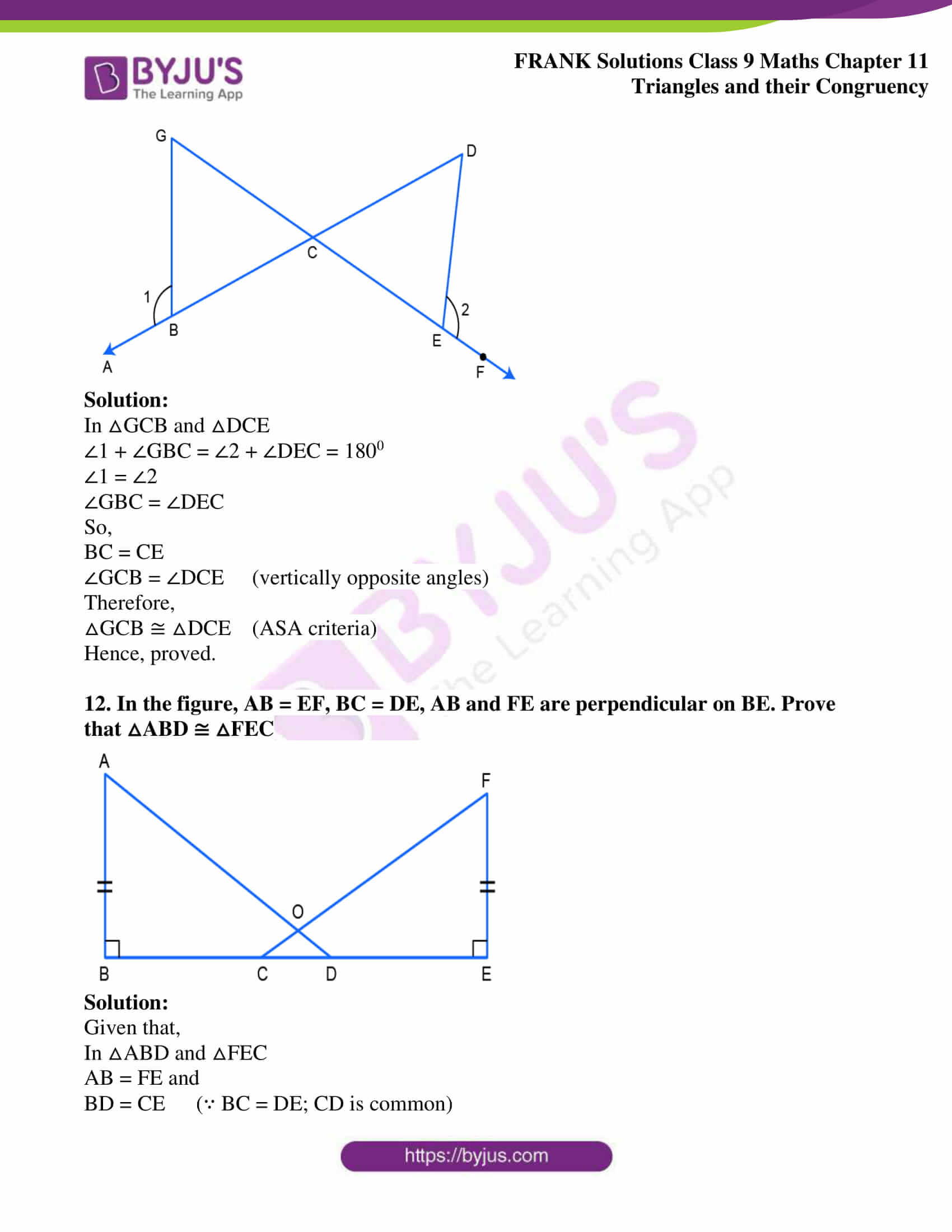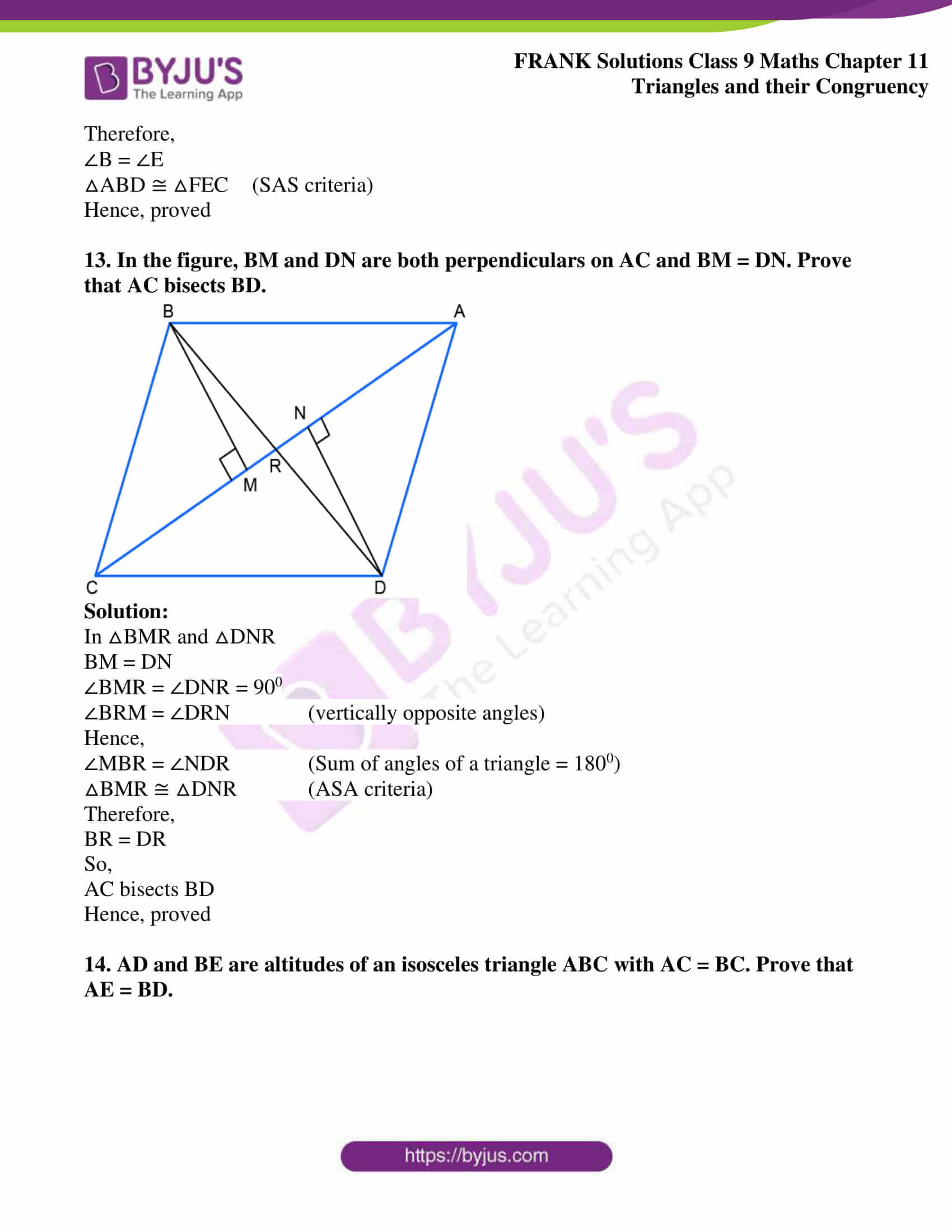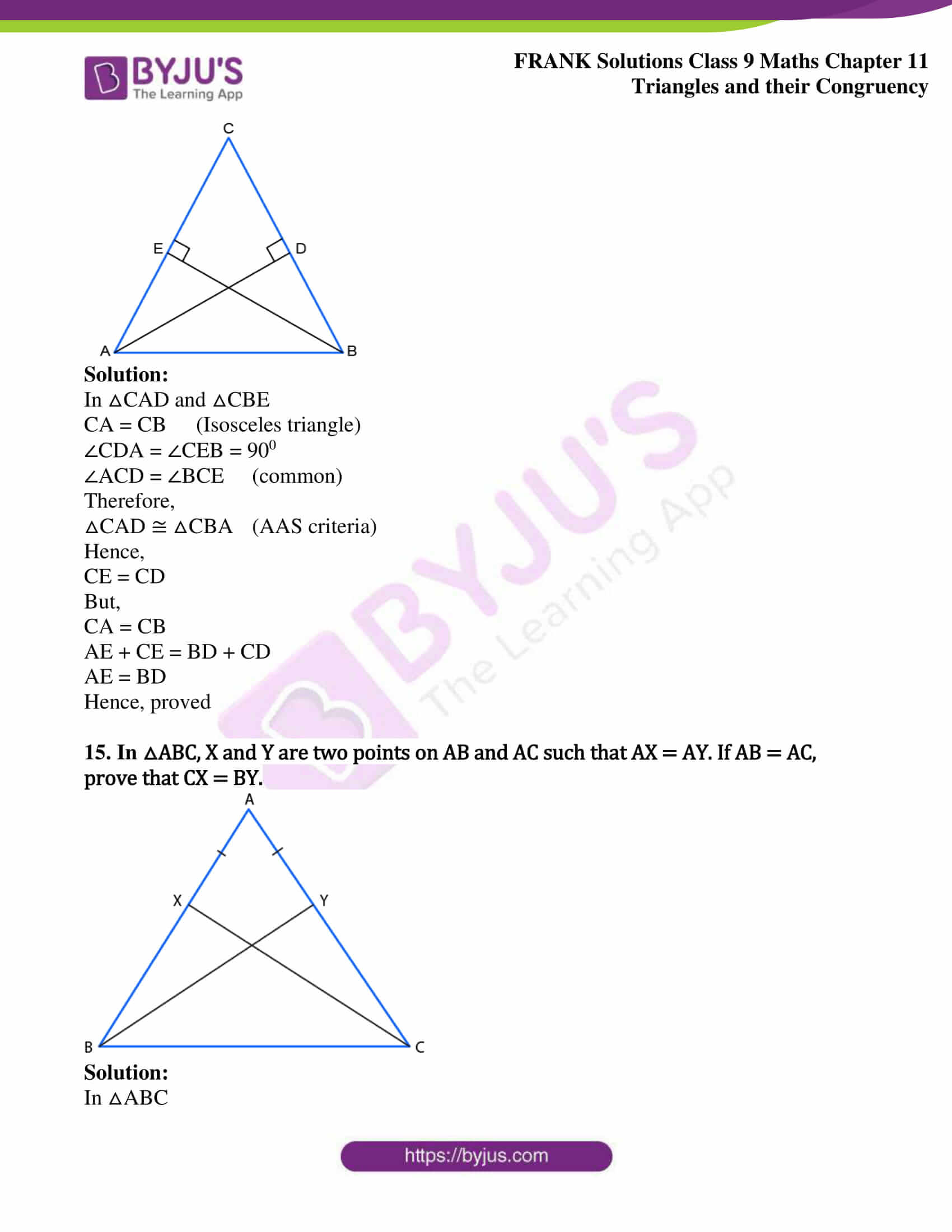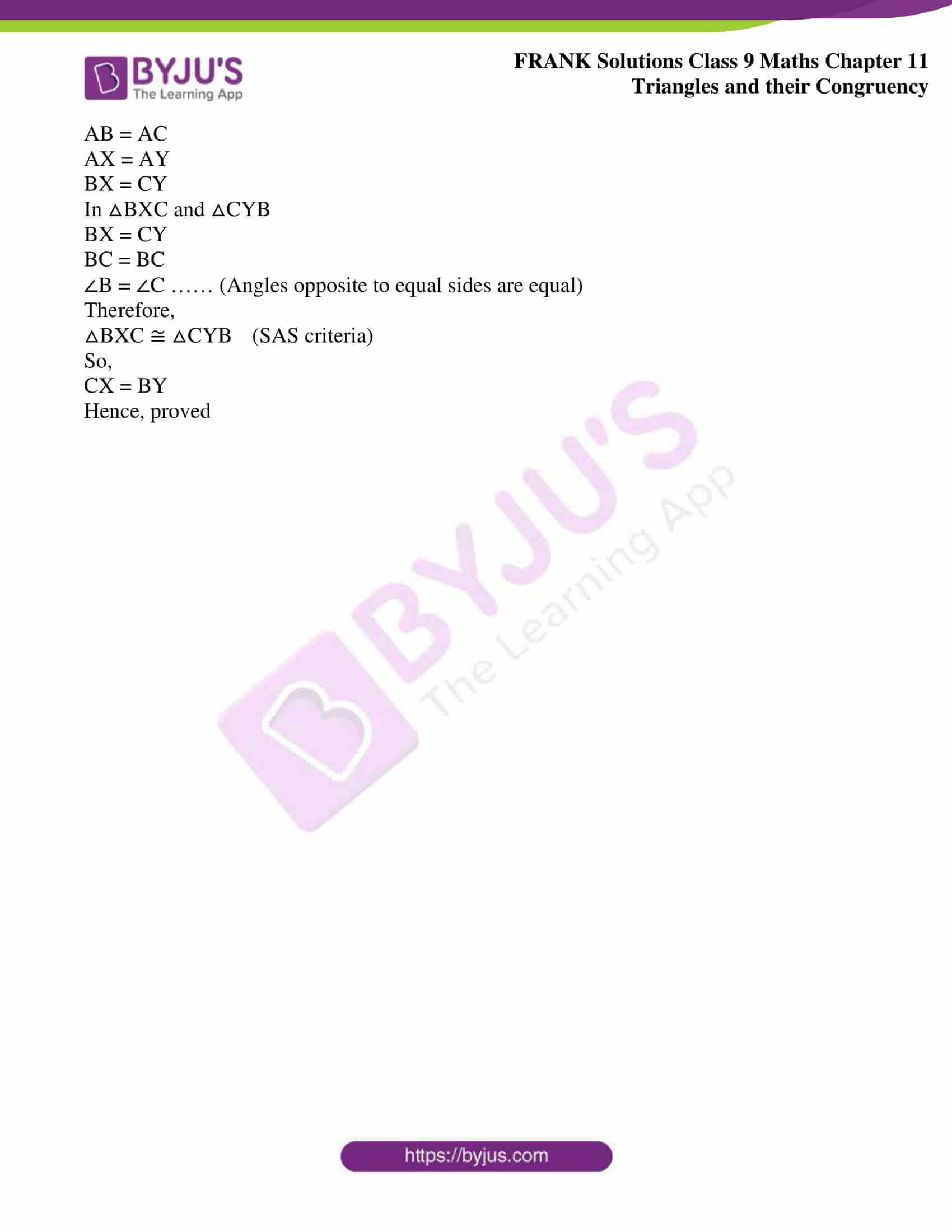## Access Frank Solutions for Class 9 Maths Chapter 11 Triangles and Their Congruency

1. In the given figure, ∠Q: ∠R = 1: 2. Find:

(a) ∠Q

(b) ∠R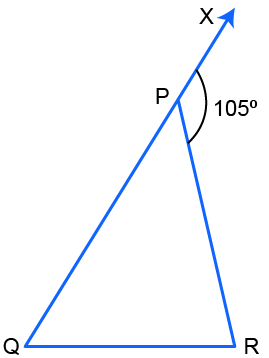Solution:Given

∠Q: ∠R = 1: 2

Let us consider ∠Q = x0

∠R = 2x0

Now,

∠RPX = ∠Q + ∠R [by exterior angle property]

1050 = x0 + 2x0

1050 = 3x0

We get,

x0 = 350

Therefore,

∠Q = x0 = 350 and

∠R = 2x0 = 700

2. The exterior angles, obtained on producing the side of a triangle both ways, are 1000 and 1200. Find all the angles of the triangle.

Solution: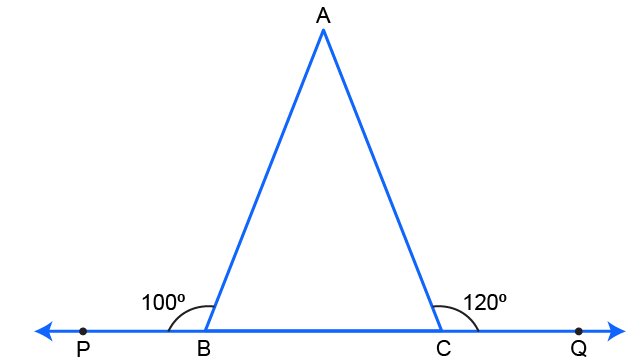∠ABP + ∠ABC = 1800 (Linear pair)

1000 + ∠ABC = 1800

∠ABC = 1800 – 1000

∠ABC = 800

∠ACQ + ∠ACB = 1800 (Linear pair)

1200 + ∠ACB = 1800

∠ACB = 1800 – 1200

∠ACB = 600

Now,

In △ABC,

∠A + ∠B + ∠C = 1800 (Angle sum property of a triangle)

∠A + 800 + 600 = 1800

∠A = 1800 – 800 – 600

We get,

∠A = 400

Therefore, the angles of a triangle are 400, 600 and 800

3. Use the given figure to find the value of x in terms of y. Calculate x, if y = 150.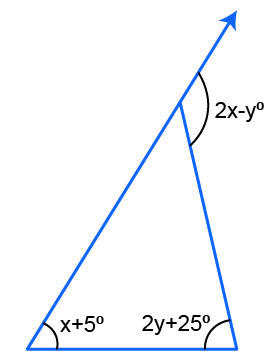Solution:(2x – y)0 = (x + 50) + (2y + 25)0 (Exterior angle property)

2x0 – y0 = x0 + 50 + 2y0 + 250

2x0 – x0 = 2y0 + y0 + 300

x0 = 3y0 + 300

When y = 150,

We have,

x0 = 3 × 150 + 300

x0 = 450 + 300

We get,

x0 = 750

4. In a triangle PQR, ∠P + ∠Q = 1300 and ∠P + ∠R = 1200. Calculate each angle of the triangle.

Solution: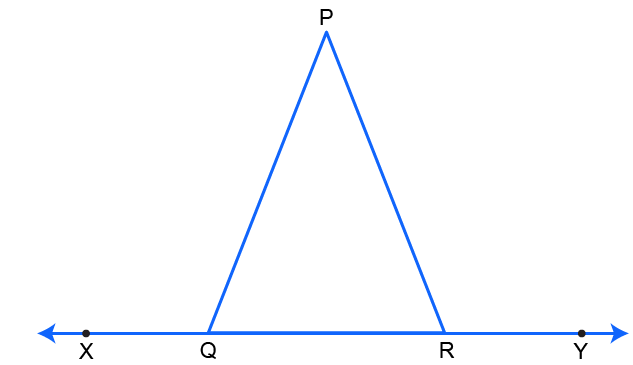Given

In △PQR,

∠P + ∠Q = 1300

WKT

∠P + ∠Q = ∠PRY (Exterior angle property)

∠PRY = 1300

∠PRY + ∠R = 1800 (Linear pair)

1300 + ∠R = 1800

∠R = 1800 – 1300

We get,

∠R = 500

Also,

Given

∠P + ∠R = 1200

Now,

∠P + ∠R = ∠PQX (Exterior angle property)

∠PQX = 1200

∠PQX + ∠Q = 1800 (Linear pair)

1200 + ∠Q = 1800

∠Q = 1800 – 1200

We get,

∠Q = 600

In △PQR,

∠P + ∠Q + ∠R = 1800 (Angle sum property of a triangle)

∠P + 600 + 500 = 1800

∠P = 1800 – 1100

We get,

∠P = 700

Therefore, the angles of △PQR are,

∠P = 700

∠Q = 600 and

∠R = 500

5. The angles of a triangle are (x + 10)0, (x + 30)0 and (x – 10)0. Find the value of ‘x’. Also, find the measure of each angle of the triangle.

Solution:

For any triangle,

Sum of measures of all three angles = 1800

Hence,

We have,

(x + 10)0 + (x + 30)0 + (x – 10)0 = 1800

x0 + 100 + x0 + 300 + x0 – 100 = 1800

3x0 + 300 = 1800

3x0 = 1800 – 300

We get,

3x0 = 1500

x0 = 500

Now,

(x + 10)0 = (50 + 10)0

(x + 10)0 = 600

(x + 30)0 = (50 + 30)0

(x + 30)0 = 800

(x – 10)0 = (50 – 10)0

(x – 10)0 = 400

Therefore, the angles of a triangle are 600, 800 and 400

6. Use the given figure to find the value of y in terms of p, q and r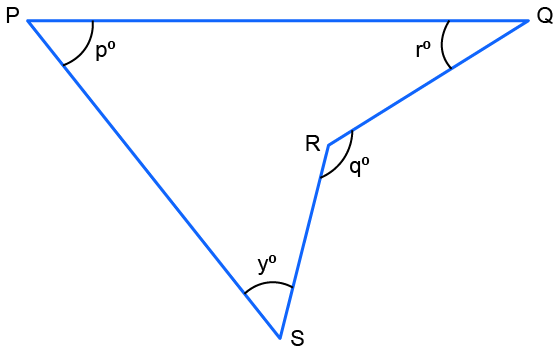Solution:

Here, SR is produced to meet PQ at point E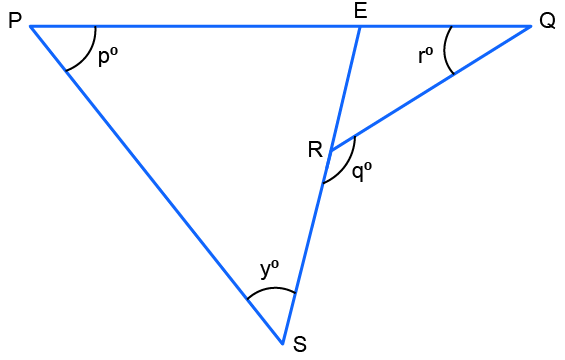In △PSE,

∠P + ∠S + ∠PES = 1800 …… (Angle sum property of a triangle)

p0 + y0 + ∠PES = 1800

∠PES = 1800 – p0 – y0 ….. (1)

In △RQE,

∠R + ∠Q + ∠REQ = 1800 …….. (Angle sum property of a triangle)

(1800 – q0) + r0 + ∠REQ = 1800

∠REQ = 1800 – (1800 – q0) – r0

∠REQ = q0 – r0 ……….(2)

Now,

∠PES + ∠REQ = 1800 ………… (Linear pair)

(1800 – p0 – y0) + (q0 – r0) = 1800 … [from (1) and (2)]

-p0 – y0 + q0 – r0 = 0

– y0 = -q0 + p0 + r0

We get,

y0 = q0 – p0 – r0

7. In the figure given below, if RS is parallel to PQ, then find the value of ∠y.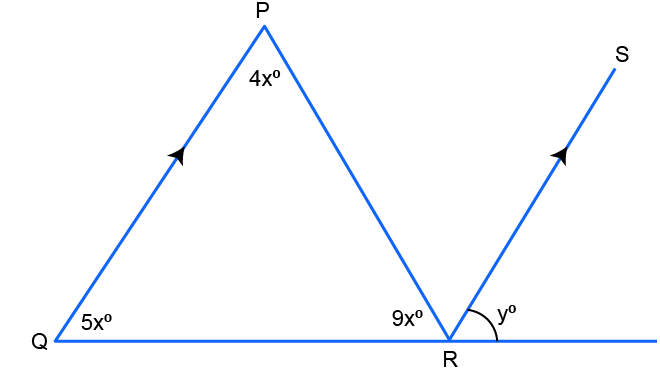In △PQR,

∠P + ∠Q + ∠R = 1800 ….. (angle sum property)

4x0 + 5x0 + 9x0 = 1800

18x0 = 1800

x = 10

∠P = 4x0 = 4 × 100

∠P = 400

∠Q = 5x0 = 5 × 100

∠Q = 500

∠QPR = ∠PRS ……. (Alternate angles)

And,

∠QPR = 400

∠PRS = 400

By exterior angle property,

∠PQR + ∠QPR = ∠PRS + y0

400 + 500 = 400 + y0

We get,

y = 500

8. Use the given figure to show that: ∠p + ∠q + ∠r = 3600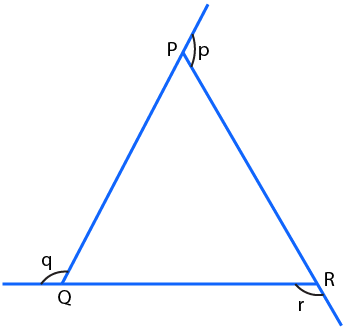Solution:

By exterior angle property,

∠p = ∠PQR + ∠PRQ

∠q = ∠QPR + ∠PRQ

∠r = ∠PQR + ∠QPR

Now,

∠p + ∠q + ∠r = ∠PQR + ∠PRQ + ∠QPR + ∠PRQ + ∠PQR + ∠QPR

On further calculation, we get,

∠p + ∠q + ∠r = 2 ∠PQR + 2∠PRQ + 2 ∠QPR

∠p + ∠q + ∠r = 2 (∠PQR + ∠PRQ + ∠QPR)

∠p + ∠q + ∠r = 2 × 1800 (Angle Sum property: ∠PQR + ∠PRQ + ∠QPR = 1800)

We get,

∠p + ∠q + ∠r = 3600

Hence,

∠p + ∠q + ∠r = 3600

9. In △ABC and △PQR, AB = PQ, BC = QR and CB and RQ are extended to X and Y, respectively and ∠ABX = ∠PQY. Prove that △ABC ≅ △PQR.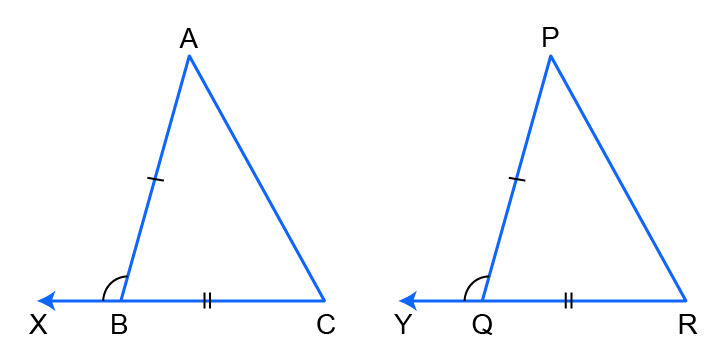Solution:

In △ABC and △PQR

AB = PQ

BC = QR

∠ABX + ∠ABC = ∠PQY + ∠PQR = 1800

So,

∠ABX = ∠PQY

∠ABC = ∠PQR

Therefore,

△ABC ≅ △PQR (SAS criteria)

Hence, proved

10. In the figure, ∠CPD = ∠BPD and AD is the bisector of ∠BAC. Prove that △CAP ≅ BAP and CP = BP.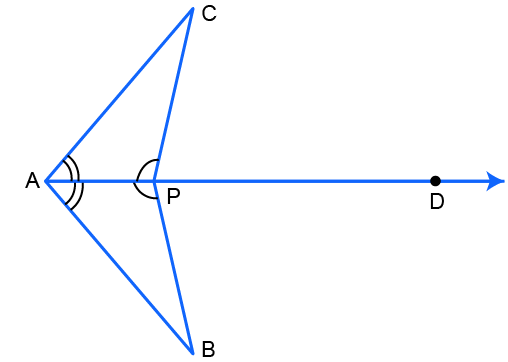Solution:

In △BAP and △CAP

∠BAP = ∠CAP (AD is the bisector of ∠BAC)

AP = AP

∠BPD + ∠BPA = ∠CPD + ∠CPA = 1800

We get,

∠BPD = ∠CPD

∠BPA = ∠CPA

Therefore,

△CAP ≅ △BAP (ASA criteria)

So,

CP = BP

Hence, proved

11. In the figure, BC = CE and ∠1 = ∠2. Prove that △GCB ≅ △DCE.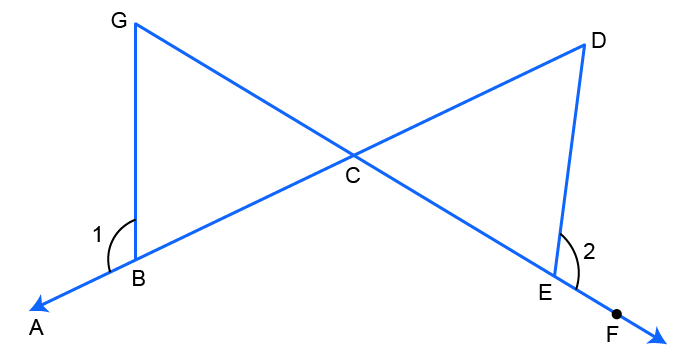Solution:

In △GCB and △DCE

∠1 + ∠GBC = ∠2 + ∠DEC = 1800

∠1 = ∠2

∠GBC = ∠DEC

So,

BC = CE

∠GCB = ∠DCE (vertically opposite angles)

Therefore,

△GCB ≅ △DCE (ASA criteria)

Hence, proved.

12. In the figure, AB = EF, BC = DE, AB and FE are perpendicular on BE. Prove that △ABD ≅ △FEC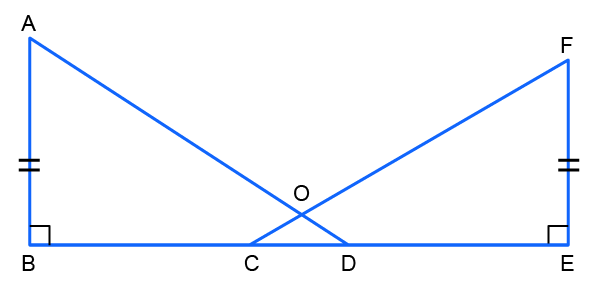Solution:

Given that,

In △ABD and △FEC

AB = FE and

BD = CE (∵ BC = DE; CD is common)

Therefore,

∠B = ∠E

△ABD ≅ △FEC (SAS criteria)

Hence, proved

13. In the figure, BM and DN are both perpendiculars on AC and BM = DN. Prove that AC bisects BD.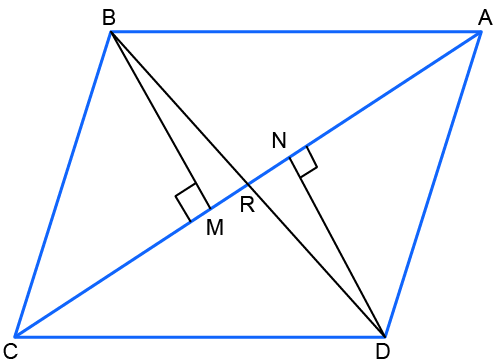Solution:

In △BMR and △DNR

BM = DN

∠BMR = ∠DNR = 900

∠BRM = ∠DRN (vertically opposite angles)

Hence,

∠MBR = ∠NDR (Sum of angles of a triangle = 1800)

△BMR ≅ △DNR (ASA criteria)

Therefore,

BR = DR

So,

AC bisects BD

Hence, proved

14. AD and BE are altitudes of an isosceles triangle ABC with AC = BC. Prove that AE = BD.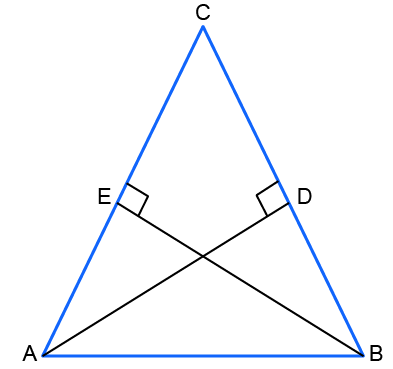Solution:

CA = CB (Isosceles triangle)

∠CDA = ∠CEB = 900

∠ACD = ∠BCE (common)

Therefore,

Hence,

CE = CD

But,

CA = CB

AE + CE = BD + CD

AE = BD

Hence, proved

15. In △ABC, X and Y are two points on AB and AC such that AX = AY. If AB = AC, prove that CX = BY.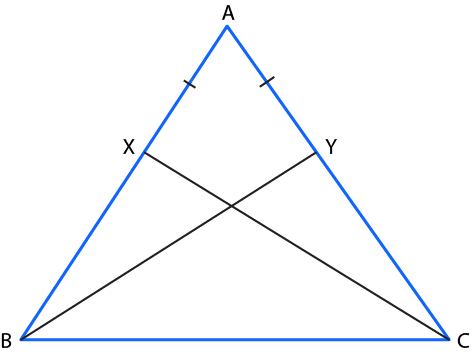Solution:

In △ABC

AB = AC

AX = AY

BX = CY

In △BXC and △CYB

BX = CY

BC = BC

∠B = ∠C …… (Angles opposite to equal sides are equal)

Therefore,

△BXC ≅ △CYB (SAS criteria)

So,

CX = BY

Hence, proved